Ex 6.3

Chapter 6 Class 6 Integers
Serial order wise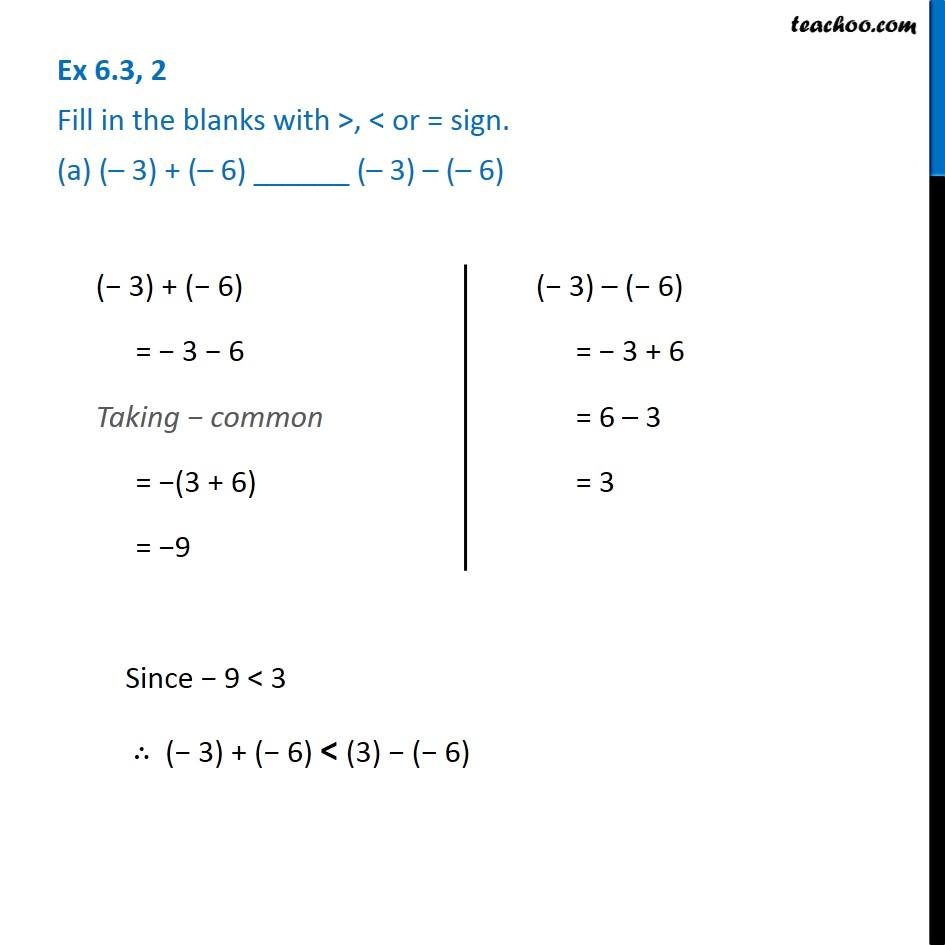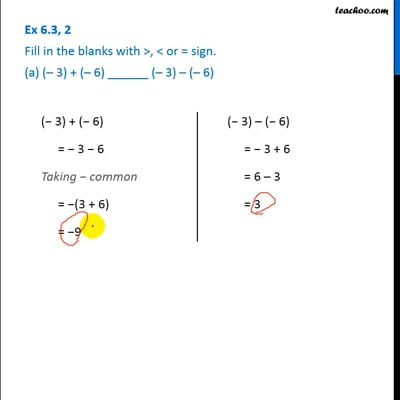This video is only available for Teachoo black users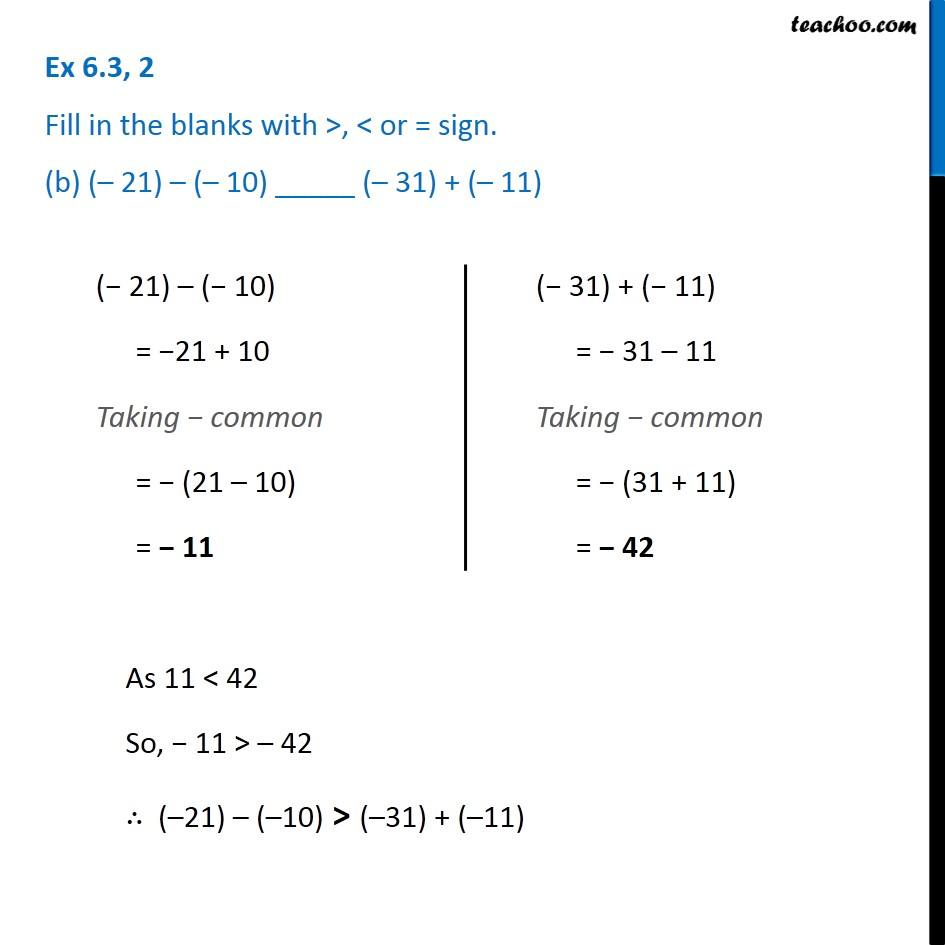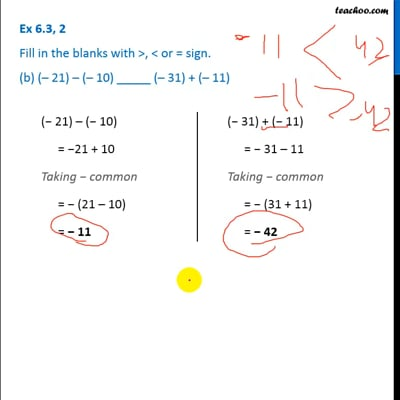This video is only available for Teachoo black users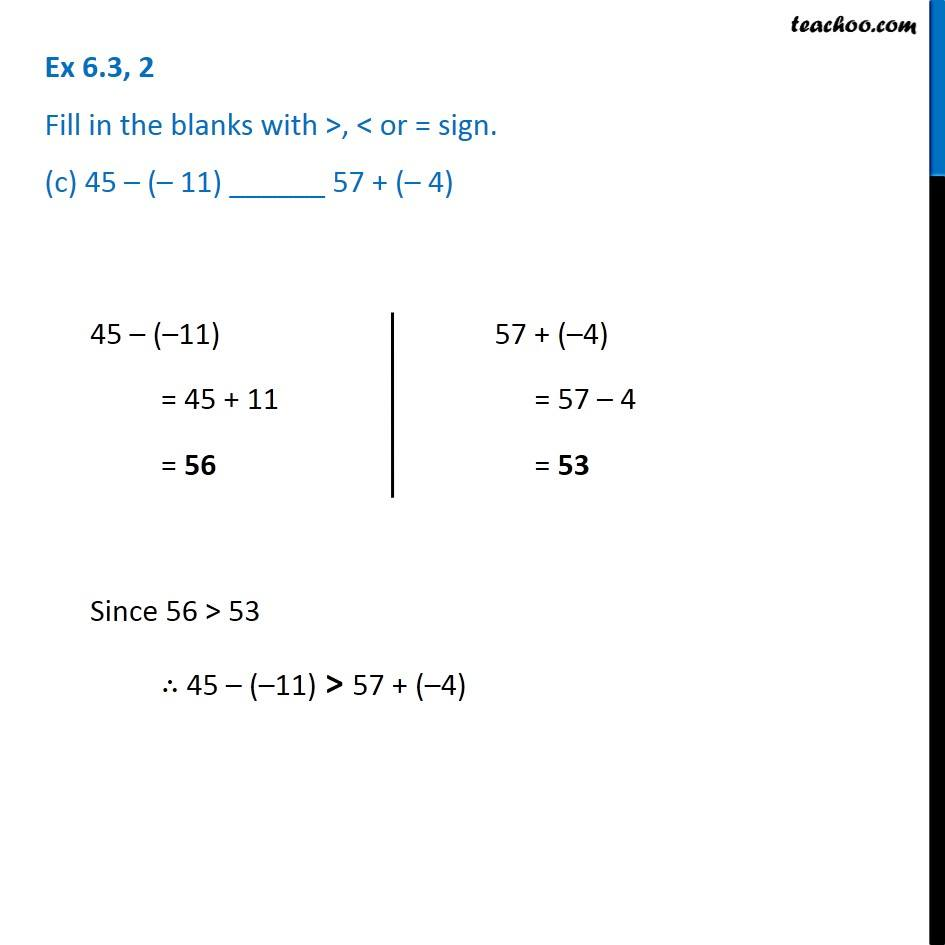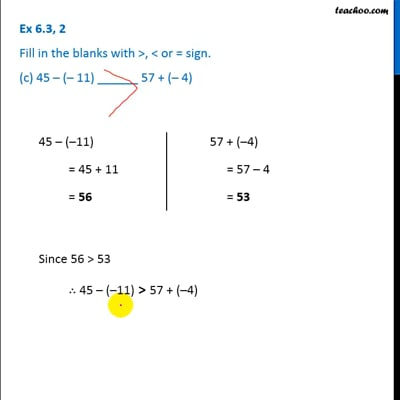This video is only available for Teachoo black users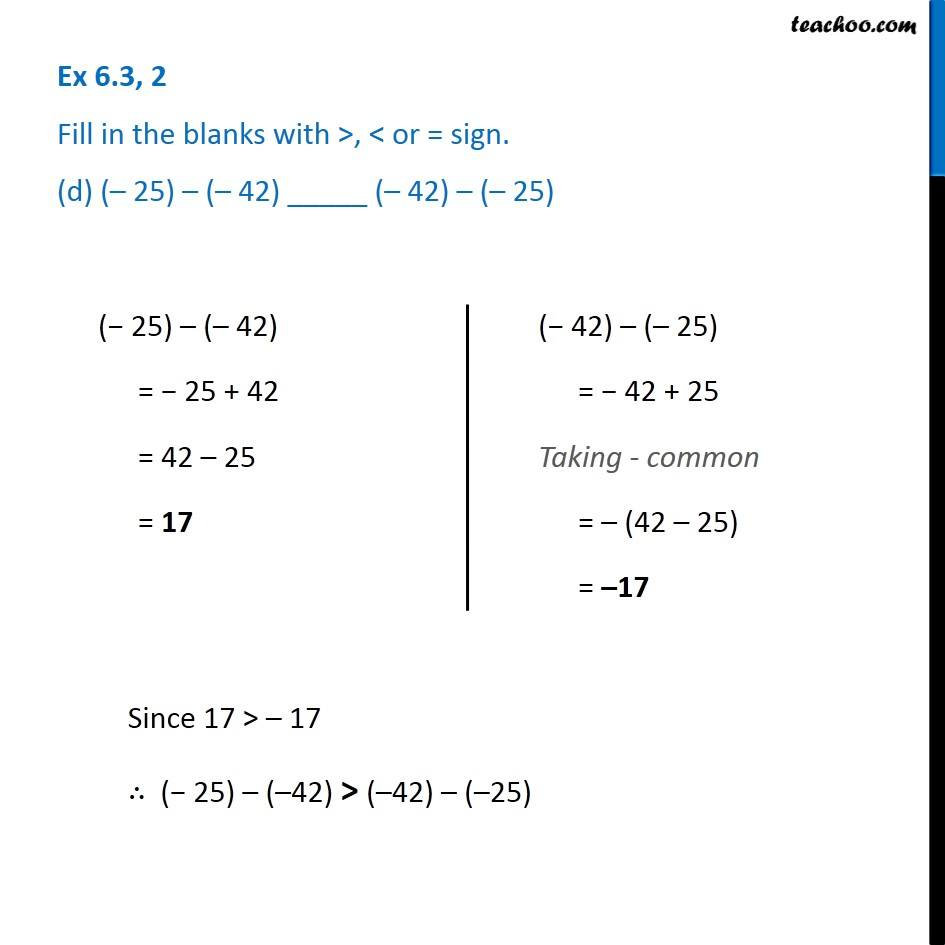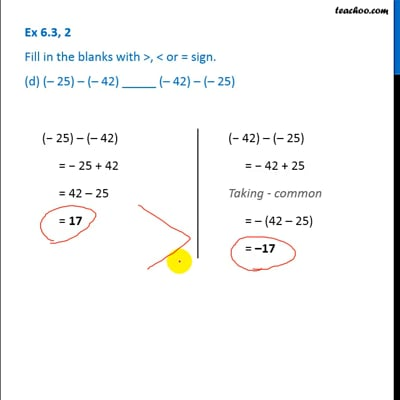This video is only available for Teachoo black users

Learn in your speed, with individual attention - Teachoo Maths 1-on-1 Class

### Transcript

Ex 6.3, 2 Fill in the blanks with >, < or = sign. (a) (– 3) + (– 6) ______ (– 3) – (– 6) (− 3) + (− 6) = − 3 − 6 Taking − common = −(3 + 6) = −9 (− 3) – (− 6) = − 3 + 6 = 6 – 3 = 3 Since − 9 < 3 ∴ (− 3) + (− 6) < (3) − (− 6) Ex 6.3, 2 Fill in the blanks with >, < or = sign. (b) (– 21) – (– 10) _____ (– 31) + (– 11) (− 21) – (− 10) = −21 + 10 Taking − common = − (21 – 10) = − 11 (− 31) + (− 11) = − 31 – 11 Taking − common = − (31 + 11) = − 42 As 11 < 42 So, − 11 > – 42 ∴ (–21) – (–10) > (–31) + (–11 Ex 6.3, 2 Fill in the blanks with >, < or = sign. (c) 45 – (– 11) ______ 57 + (– 4) 45 – (–11) = 45 + 11 = 56 57 + (–4) = 57 – 4 = 53 Since 56 > 53 ∴ 45 – (–11) > 57 + (–4) Ex 6.3, 2 Fill in the blanks with >, < or = sign. (d) (– 25) – (– 42) _____ (– 42) – (– 25) (− 25) – (– 42) = − 25 + 42 = 42 – 25 = 17 (− 42) – (– 25) = − 42 + 25 Taking - common = – (42 – 25) = –17 Since 17 > – 17 ∴ (− 25) – (–42) > (–42) – (–25)